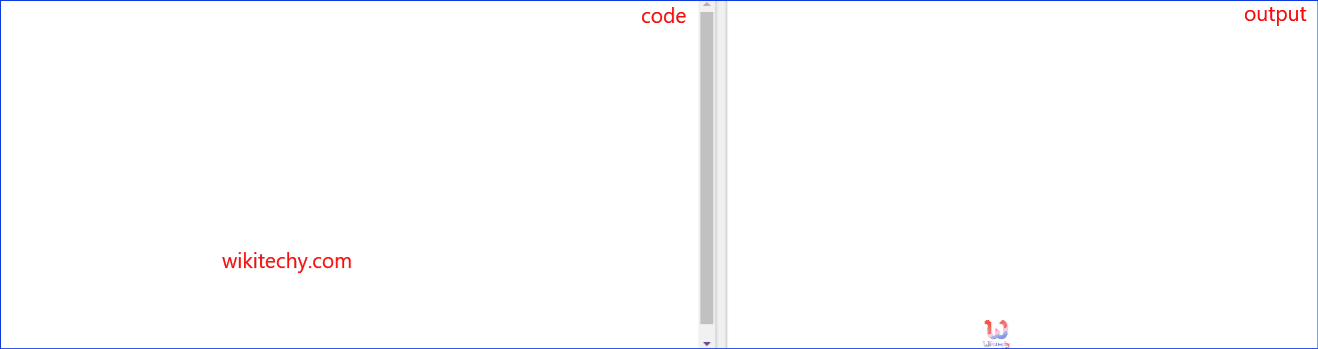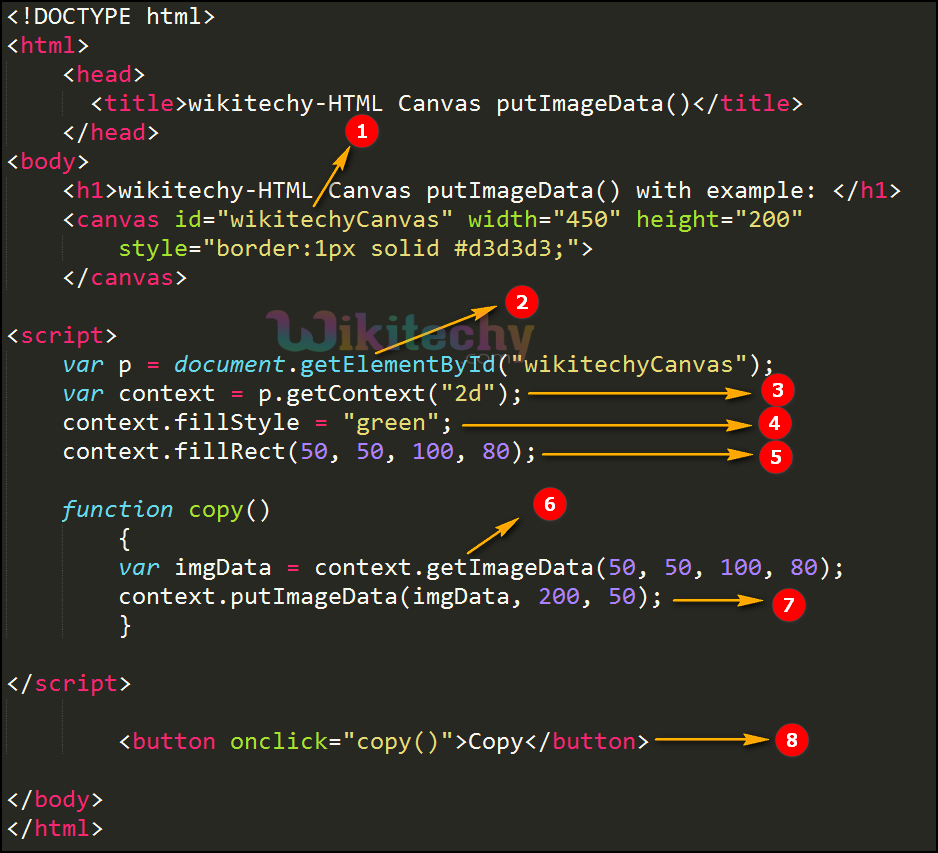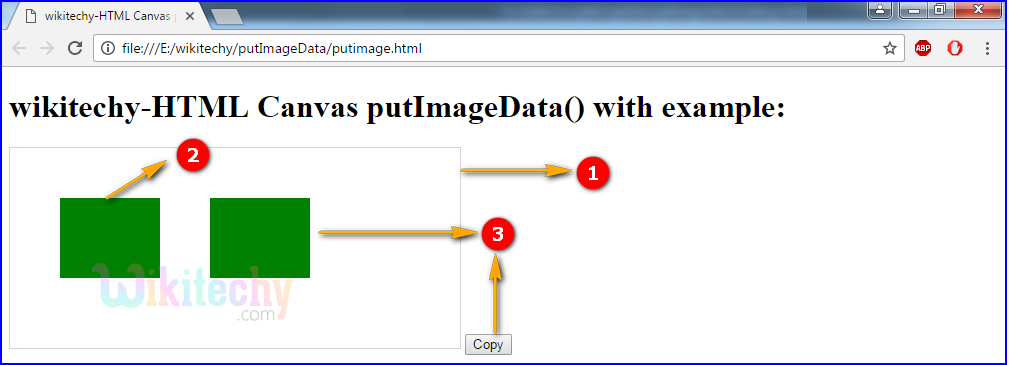# html tutorial - putImageData() Method in HTML5 Canvas - html5 - html code - html formLearn html - html tutorial - Putimagedata method in html5 canvas - html examples - html programs

• The putImageData() method is one of the canvas method.
• This method is used to puts the image data (from a specified ImageData object) back onto the canvas.

## Parameter values for putImageData() Method in HTML5 Canvas:

Parameter Description
imgData To specifies imageData object to put back on the canvas.
x To start copy from upper left corner the x-coordinate in pixels.
y To start copy from upper left corner the y-coordinate in pixels
dirty x The horizontal (x) value is used, where to place the image on the canvas in pixels.
dirty y The vertical (y) value is used, where to place the image on the canvas in pixels.
dirtyWidth The width is used to draw the image on the canvas.
dirtyHeight The height is used to draw the image on the canvas.

## Code Explanation for putImageData() Method in HTML5 Canvas:1. ”wikitechyCanvas” is used to declare the id value of the canvas tag.
2. getElementById: method is used to draw the element from canvas id value.
3. getContext(): returns an object that provides methods and properties for drawing on the canvas.
4. The fillStyle property is used to fill the green color in rectangle.
5. To create a rectangle the value is set as (50, 50, 100, 80) in (x,y,width,height) axis.
6. The getImageData(50, 50, 100, 80) is used to copy the above rectangle.
7. The image will put back on the canvas using putImageData (imgData,200 ,50 ).
8. button is used to copy the rectangle.

## Output for putImageData() Method in HTML5 Canvas:1. <canvas> is used to draw a rectangle with gray color border.
2. canvas is used to draw a rectangle an putImageData() method.
3. Here the output is displayed by green color rectangle.
-The first rectangle is displayed by (50, 50, 100, 80) in (x,y,width,height) axis.
4. The putImageData(imgData,200 ,50 ) is used for copy the rectangle and display the image back on the canvas.

## Browser Support for putImageData() Method in HTML5 Canvas:Yes 9.0 Yes Yes Yes

## Tips and Notes

• The getImageData() methodis used to copies the pixel data for a specified rectangle on a canvas.
• The createImageData() method is used to creates a new, blank ImageData object.